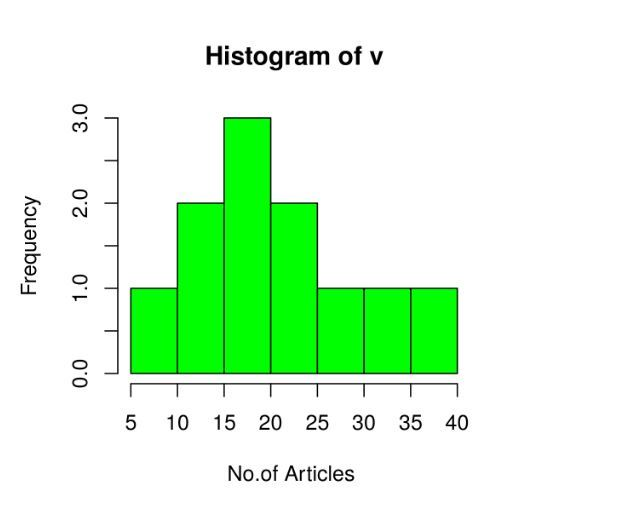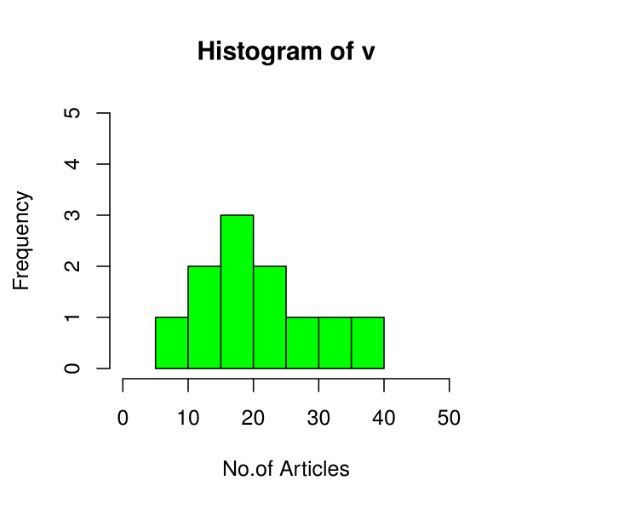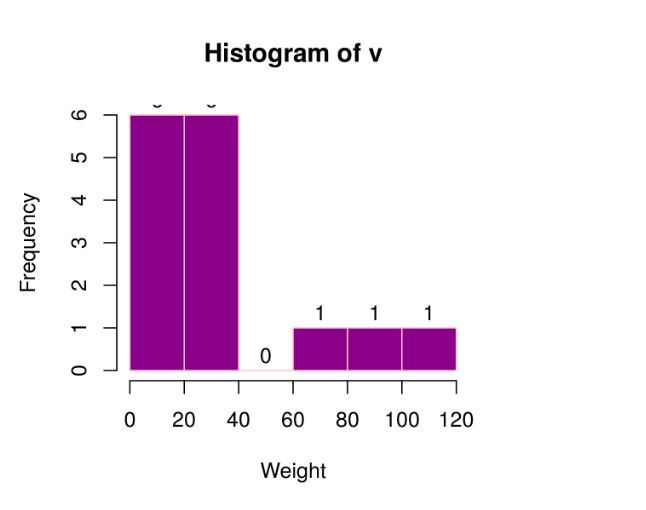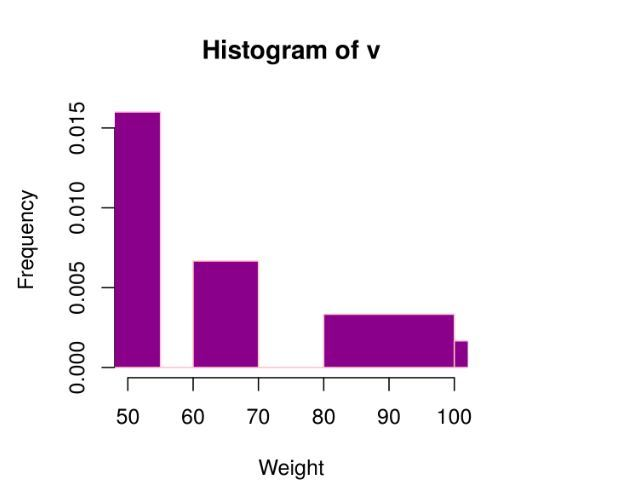Open in App
Not now

# Histograms in R language

• Last Updated : 09 Dec, 2021

A histogram contains a rectangular area to display the statistical information which is proportional to the frequency of a variable and its width in successive numerical intervals. A graphical representation that manages a group of data points into different specified ranges. It has a special feature which shows no gaps between the bars and is similar to a vertical bar graph.

## R – Histograms

We can create histogram in R Programming Language using hist() function.

Syntax: hist(v, main, xlab, xlim, ylim, breaks, col, border)

Parameters:

• v: This parameter contains numerical values used in histogram.
• main: This parameter main is the title of the chart.
• col: This parameter is used to set color of the bars.
• xlab: This parameter is the label for horizontal axis.
• border: This parameter is used to set border color of each bar.
• xlim: This parameter is used for plotting values of x-axis.
• ylim: This parameter is used for plotting values of y-axis.
• breaks: This parameter is used as width of each bar.

### Creating a simple Histogram in R

Creating a simple histogram chart by using the above parameter. This vector v is plot using hist().

Example:

## R

 `# Create data for the graph.` `v <- ``c``(19, 23, 11, 5, 16, 21, 32, ` `       ``14, 19, 27, 39)`   `# Create the histogram.` `hist``(v, xlab = ``"No.of Articles "``,` `     ``col = ``"green"``, border = ``"black"``)`

Output:### Range of X and Y values

To describe the range of values we need to do the following steps:

1. We can use the xlim and ylim parameter in X-axis and Y-axis.
2. Take all parameters which are required to make histogram chart.

Example

## R

 `# Create data for the graph.` `v <- ``c``(19, 23, 11, 5, 16, 21, 32, 14, 19, 27, 39)`     `# Create the histogram.` `hist``(v, xlab = ``"No.of Articles"``, col = ``"green"``,` `    ``border = ``"black"``, xlim = ``c``(0, 50),` `    ``ylim = ``c``(0, 5), breaks = 5)    `

Output:### Using histogram return values for labels using text()

To create a histogram return value chart.

## R

 `# Creating data for the graph.` `v <- ``c``(19, 23, 11, 5, 16, 21, 32, 14, 19,` `       ``27, 39, 120, 40, 70, 90)`   `# Creating the histogram.` `m<-``hist``(v, xlab = ``"Weight"``, ylab =``"Frequency"``,` `        ``col = ``"darkmagenta"``, border = ``"pink"``, ` `        ``breaks = 5)`   `# Setting labels` `text``(m\$mids, m\$counts, labels = m\$counts, ` `     ``adj = ``c``(0.5, -0.5))    `

Output:### Histogram using non-uniform width

Creating different width histogram charts, by using the above parameters, we created histogram using non-uniform width.

Example

## R

 `# Creating data for the graph.` `v <- ``c``(19, 23, 11, 5, 16, 21, 32, 14,` `       ``19, 27, 39, 120, 40, 70, 90)` `    `  `# Creating the histogram.` `hist``(v, xlab = ``"Weight"``, ylab =``"Frequency"``,` `     ``xlim = ``c``(50, 100),` `    ``col = ``"darkmagenta"``, border = ``"pink"``,` `    ``breaks = ``c``(5, 55, 60, 70, 75,` `               ``80, 100, 140))`

Output:My Personal Notes arrow_drop_up
Related Articles